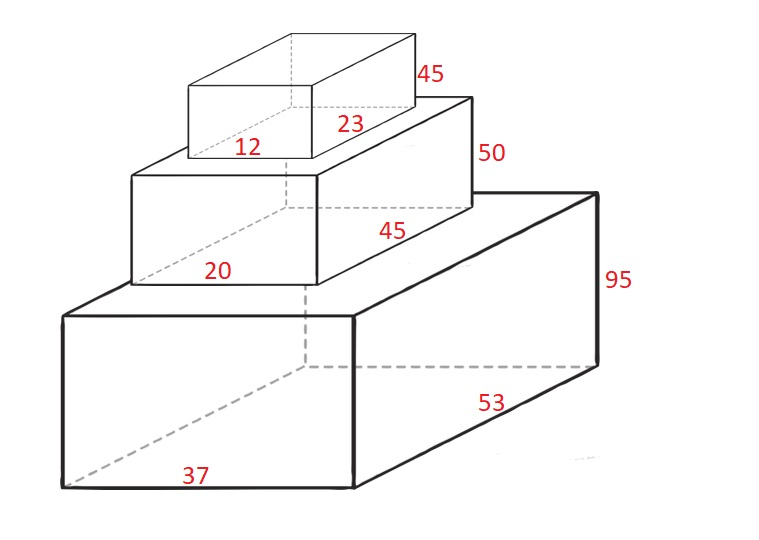Formatted question description: https://leetcode.ca/all/1691.html

# Question

Given n cuboids where the dimensions of the i-th cuboid is cuboids[i] = [width_i, length_i, height_i] (0-indexed). Choose a subset of cuboids and place them on each other.

You can place cuboid i on cuboid j if width_i <= width_j and length_i <= length_j and height_i <= height_j. You can rearrange any cuboid’s dimensions by rotating it to put it on another cuboid.

Return the maximum height of the stacked cuboids.

Example 1:Input: cuboids = [[50,45,20],[95,37,53],[45,23,12]]

Output: 190

Explanation:

Cuboid 1 is placed on the bottom with the 53x37 side facing down with height 95.

Cuboid 0 is placed next with the 45x20 side facing down with height 50.

Cuboid 2 is placed next with the 23x12 side facing down with height 45.

The total height is 95 + 50 + 45 = 190.

Example 2:

Input: cuboids = [[38,25,45],[76,35,3]]

Output: 76

Explanation:

You can’t place any of the cuboids on the other.

We choose cuboid 1 and rotate it so that the 35x3 side is facing down and its height is 76.

Example 3:

Input: cuboids = [[7,11,17],[7,17,11],[11,7,17],[11,17,7],[17,7,11],[17,11,7]]

Output: 102

Explanation:

After rearranging the cuboids, you can see that all cuboids have the same dimension.

You can place the 11x7 side down on all cuboids so their heights are 17.

The maximum height of stacked cuboids is 6 * 17 = 102.

Constraints:

• n == cuboids.length
• 1 <= n <= 100
• 1 <= width_i, length_i, height_i <= 100

## Solution

First, rearrange all the cuboids such that for any cuboids[i], there is cuboids[i] <= cuboids[i] <= cuboids[i]. Then sort all the cuboids according to their widths, lengths and heights.

For any two cuboids cuboids[j] and cuboids[i] where 0 <= j < i < n, cuboids[j] can be placed on cuboids[i] if and only if cuboids[j] <= cuboids[i] and cuboids[j] <= cuboids[i] and cuboids[j] <= cuboids[i]. If this is not the case, then cuboids[j] cannot be placed on cuboids[i] even if the two cubiods are rearranged.

After all the cuboids are rearranged and sorted, use dynamic programming to find the maximum height. Create an array dp of length n such that dp[i] represents the maximum height when cuboids[i] is used. Initially, dp[i] = cuboids[i] for all 0 <= i < n. For each cuboid, always use the last dimention since it is the dimension with the maximum value in the cuboid. For each 1 <= i < n, loop over 0 <= j < i. If cuboids[j] can be placed on cuboids[i], then update dp[i] using dp[j] + cuboids[i].

In the dynamic programming process, maintain the maximum height. Finally, return the maximum height.

This question is related to 354 Russian Doll Envelopes, expanding from a 2-D issue to 3-D issue.

# Code

## Java version

Java

class Solution {
public int maxHeight(int[][] cuboids) {
for (int[] cuboid : cuboids)
Arrays.sort(cuboid);
Arrays.sort(cuboids, new Comparator<int[]>() {
public int compare(int[] cuboid1, int[] cuboid2) {
if (cuboid1 != cuboid2)
return cuboid1 - cuboid2;
else if (cuboid1 != cuboid2)
return cuboid1 - cuboid2;
else
return cuboid1 - cuboid2;
}
});
int maxHeight = 0;
int cuboidsCount = cuboids.length;
int[] dp = new int[cuboidsCount];
for (int i = 0; i < cuboidsCount; i++) {
int height = cuboids[i];
maxHeight = Math.max(maxHeight, height);
dp[i] = height;
}
for (int i = 1; i < cuboidsCount; i++) {
int width = cuboids[i], length = cuboids[i], height = cuboids[i];
for (int j = i - 1; j >= 0; j--) {
if (cuboids[j] <= width && cuboids[j] <= length && cuboids[j] <= height) {
int totalHeight = dp[j] + height;
maxHeight = Math.max(maxHeight, totalHeight);
dp[i] = Math.max(dp[i], totalHeight);
}
}
}
return maxHeight;
}
}